<< Back to Off-topic Forum .GrayedOutTextBox { color: Gray; }

 Posts 1 - 15 of 15
Help with calculus: 11/2/2016 00:03:31Shredtail2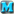Level 57
Report
So my latest homework involves the Mean Value Theorem. I feel that it wasn't explained very well in class today, and I don't really know what the heck I'm doing.

Also, I'm stuck on questions relating to certain derivatives. I know that d/dx means to find the derivative as normal, but how does dy/dx differ from d/dx? I'm rather stumped now and I've failed my past couple of tests in the class as a result of being confused (latest test, I got a 55 on). Can anyone explain that to me? It would be much appreciated. Thank you.
Help with calculus: 11/2/2016 00:11:29Rogue NK
Level 59
Report
d/dx is the derivative with respect to x.

dy/dx is the derivative with respect to y.

If you have any further questions I have made it to Calc 3.
Help with calculus: 11/2/2016 00:13:10Shredtail2Level 57
Report
Thank you very much, I'll watch the video. What would be the differences between calculating d/dx and dy/dx? How would I approach the problem differently?

Edited 11/2/2016 00:13:46
Help with calculus: 11/2/2016 00:15:22Hostile
Level 58
Report
y = x + 1

dy/dx = d/dx * (X+1)

It is basically substitution.

dy/dx is the Leibniz notation of the derivative.

You need two deltas something to do stuff.

Edited 11/2/2016 00:16:06
Help with calculus: 11/2/2016 00:20:31Shredtail2Level 57
Report
Thanks. That helped.
Help with calculus: 11/2/2016 17:15:19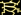Farah♦Level 60
Report
d/dx is the derivative with respect to x.

dy/dx is the derivative with respect to y.
Making it to calc III while thinking this is true?

dy/dx means you're taking the derivative of the function y with respect to x.
d/dx means you're taking the derivative of whatever you write after it.
Help with calculus: 11/2/2016 19:50:58Hostile
Level 58
Report
You need to do the derivative of x and y in dy/dx.

(x stuff)' = (x stuff * dx)

(y stuff)' = (y stuff * dy)

You apply the same operations in both sides of the equations.

If you have y = (x + 1), you do y' = (x + 1)'.
It gives 1 dy = 1 dx.
dy/dx = 1.

Example :

y meters = x seconds => meters / second
y meters / seconds = x seconds => meters / second squared
Help with calculus: 11/2/2016 20:23:41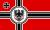Leibstandarte (Vengeance)
Level 45
Report
1+1 = 11?
Help with calculus: 11/2/2016 20:47:15Hostile
Level 58
Report
1 + 1 = 1

http://mathforum.org/library/drmath/view/57110.html

Proven in the good old 90s (tm).
Help with calculus: 11/4/2016 09:15:48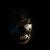Level 50
Report
The Mean Value Theorem regarding derivatives, states that for at least one point over a closed interval a tangent line at that point will be parallel to a secant line draw through the end points of the interval. The function must be continuous over the closed interval and differentiable over the open interval. The meaning of this statement becomes clear when you think about it geometrically. That is to say if you draw the function in question, and you draw a line (secant) between the end points of the interval which you are interested in, it must be the case that a tangent (to the function which is continuous over the interval in question) parallel to the drawn line must exist.

The derivative is the slope of the function.

Take a look at the Wikipedia MVT page.

Additionally, patrickjmt has many good calculus tutorials on YouTube. His video's are a nice option if you don't care for Salman Kahn.
Help with calculus: 11/4/2016 10:27:57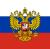Remove Kebab
Level 58
Report
Get extra help after school.
Help with calculus: 11/4/2016 11:09:13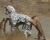Hades
Level 63
Report
Help with calculus: 11/4/2016 11:31:30Hostile
Level 58
Report
Gradients are things used in hydraulics.

Too complicated for me. :(
Help with calculus: 11/4/2016 19:52:17Aura GuardianLevel 62
Report
d/dx[some funtion] means to take the derivative with respect to x.
dy/dx = [Some function] means that [Some function] is the derivative of y(x)

Say, for example, you want to take the derivative of x^2:

y(x) = x^2

you would evaluate:

d/dx[x^2]

To find:

dy/dx = 2x

The mean value theorem states that if you draw a curve on a graph, pick any two points, and draw a secant line, the slope of that secant line will be equal to, at at least one point, of the slope of the curve at one point within the x-values of those two points (the interval), given that the graph is continuous with no sharp corners.

Taking y(x) = x^2 again, say that you take x = 0 and x = 1

you find the points on the function to be (0,0) and (1,1).

Use the slope between two points equation, (y_2 - y_1)/(x_2 - x_1) to find the slope of the secant line between the points (0,0) and (1,1) to be 1.

Since we used x = 0 and x = 1, the interval is (0, 1) or [0, 1], it could be either, (this is actually a rather hotly debated topic in calculus)

According to mean value theorem, there must be a specific point where the slope of y(x) = x^2 ITSELF is equal to 1, between x = 0 and x = 1, since y(x) = x^2 is continuous and has NO sharp corners.

What equation gives us the slope of a specific point on a function? the derivative, of course.

The derivative of y(x) = x^2 is 2x.

2x = 1 (setting the derivative equal to the secant value)

x = 1/2 (this is where the slope of the function is equal to 1)

Since 1/2 is in between the values of 0 and 1, we have verified the mean value theorem for this case.

Edited 11/4/2016 20:13:11
Help with calculus: 11/4/2016 20:31:52Shredtail2Level 57
Report
Thank you guys once again. I got it figured out, and managed to work my grade up to a 90 with a combination of tutorials and the quick help I got here. I really and truly appreciate it.
 Posts 1 - 15 of 15